2013-07-11 17:54:37 saintfox001 阅读数 3963
• ###### MATLAB图像处理

全面系统的学习MATLAB在图像处理中的应用

20788 人正在学习 去看看 魏伟

——（完整工程文件到我的资源下载）

1.图像中值滤波的背景和意义

2.中值滤波的原理方法

n为偶数时，y=x(k1)+x(k2),k1=n/2,k2=k1+1。

3*3的模板，对9个数排序，取第5个数替代原来的像素值。以某一个像素点为中心，选取一个3*3的窗口为模版，如图11-2-3：模版中的灰度值依次为｛2,1,4,2,2,3,7,6,8｝，排序后新的灰度值为｛1,2,2,2,3,4,6,7,8｝,第五个数3为排序后的中值，用3取代原来的中心值2，完成中值滤波过程。1选择一个(2n+1)×(2n+1)的窗口(通常为3×3或5×5)，并用该窗口沿图像数据进行行或列方向的移位滑动；

2 每次移动后，对窗内的诸像素灰度值进行排序；

3 用排序所得中值替代窗口中心位置的原始像素灰度值。

3.实验的C语言实现代码

/*========  头文件引用===========*/

#include "stdio.h"

#include "math.h"

/*============= 工作变量定义======*/

unsigned char *pr_n;    //指针定义

unsigned char *pr_s;    //指针定义

//说明：定义数据存放变量

#pragma        DATA_SECTION(IMG,"data");

int  IMG;

#pragma        DATA_SECTION(Noise_IMG,"data");

unsigned char  Noise_IMG;

#pragma        DATA_SECTION(Smooth_IMG,"data");

unsigned char  Smooth_IMG;

void  Medel_Smooth();

///////////////////////////////////////////////////////////////////////////////////////////////////////////////

//使用说明：

//   1. 本程序可以在Simulator下运动;

//   2. 程序编译、链接、加载成功后，先

//     （说明：*.dat格式，内部存放了某图像各像素的灰度值）加载入数据存储器存储地址Noise_IMG中

//   3. 数据加载成功后，再Debug/Go Main, 一步一步运行程序

///////////////////////////////////////////////////////////////////////////////////////////////////////////////

/*================= 主程序 ================*/

main()

{

long n;

int imgH,imgW;

int *ptr;

imgH=160;  //图像高与宽，因为数据文件中的图像是160X160像素的

imgW=160;

/*=========== 初始化 ==========*/

//1 把图像数据从IMG中移到Noise_IMG数组中

ptr=IMG;

pr_n=Noise_IMG;

for (n=0;n<imgH*imgW;n++)

*pr_n++=*ptr++;

//说明：在此暂停，可看到噪声图像

//指针指向数组

pr_n=Noise_IMG;

pr_s=Smooth_IMG;

//2 调用子程序，进行彩色图像变换成灰度图像

while (1)

{

Medel_Smooth(pr_n,pr_s,imgW,imgH);

//说明：上面子程序执行后，在此暂停，可看平滑后的图像

}

//说明：在此暂停，可看变换后的灰度图像

}

/*============== 子程序 =============*/

void Medel_Smooth

(   unsigned char *sourceImg,     //原始图像

unsigned char *newImg,       //输出：中值滤波后图像

int cols, int rows           //图像的宽度与高度     /* 图像的宽度与高度            */

)

{     //定义局部变量

int i,j,k,m,r,range=32;

int pixels;

unsigned char *pp=sourceImg, *newpp=newImg;

int medianWin={0};

int temp;

for (i=0; i< cols -1; i++)

*newpp++ = *pp++;

pp  =  sourceImg + (rows-1)* cols;

newpp =  newImg+ (rows-1)* cols;

for (i=0; i< cols -1; i++)

*newpp++ = *pp++;

newpp  =  newImg;

pp     =  sourceImg;

for (i=0; i< rows -1; i++)

{           *newpp = *pp;

newpp+=cols; pp+=cols;

}

newpp  =  newImg+cols-1;

pp     =  sourceImg+cols-1;

for (i=0; i< rows -1; i++)

{

*newpp = *pp;

newpp+=cols; pp+=cols;

}

pixels=(cols-2)*(rows-2);

pp=sourceImg+cols+1;

newpp=newImg+cols+1;

for(i=0;i<pixels;i++)

{

medianWin=*(pp - cols-1);

medianWin=*(pp -cols);

medianWin=*(pp - cols+1);

medianWin=*(pp-1);

medianWin=*pp;

medianWin=*(pp+1);

medianWin=*(pp + cols-1);

medianWin=*(pp + cols);

medianWin=*(pp + cols+1);

r=8;

for(k=0;k<r;k++,r--)

for(m=0;m<r;m++)

{

{if ( medianWin[m]>medianWin[m+1] )

{temp=medianWin[m];

medianWin[m]=medianWin[m+1];

medianWin[m+1]=temp;

}

}

}

*newpp=medianWin; //排序后取中间值赋值*newpp

newpp++;

pp++; }            }//程序结束

4.实验步骤与结果

1实验设备

1. PC兼容机一台：操作系统为Windows XP (Windows NT、Windows 98、Windows 2000)，Windows操作系统的内核如果是NT的英安装相应的补丁程序(如：Windows 2000为Service Pack3，Windows XP为 Service Pack1)。

2. Code Composer Studio 2.2 软件开发环境。

3. TS-DM64x实验箱一台（软件仿真可以不要实验箱）。

2实验步骤

1设置ccs为软件仿真环境2. 启动CCS。

3. 打开工程，编译并运行程序。

4. 运行程序，观察试验结果。

（1）编译、链接程序：执行菜单Project/Rebuild All，汇编结果在将汇编信息输出窗口中给出。编译后将在Bebug目录中产生一个ImgSmooth.out文件。（4）运行程序：执行Debug/Run。为了便于观看试验前后的结果，可以在程序中设置断点，采用单步执行的方法运行程序。

（5）显示平滑前的噪声图像：执行View/Graph/Image，在弹出的对话框中选择颜色类型为RGB，并输入RGB彩色图像三个通道数据的地址，以及图像显示格式（显示几行、每行几像素）等内容，如下图所示。这样，原始的噪声图像如图1所示。

（6）显示平滑后的图像：执行View/Graph/Image，在弹出的对话框中选择颜色类型为RGB，并输入灰度图像据的地址，以及图像显示格式（显示几行、每行几像素）等内容，如下图所示。这样平滑后的图像如图2(7)按照上述方法加载带有椒盐噪声的图像，并进行中值滤波，观察实验结果。如3图所示5.实验结果分析与总结

2017-04-06 17:38:07 oHanTanYanYing 阅读数 3549
• ###### MATLAB图像处理

全面系统的学习MATLAB在图像处理中的应用

20788 人正在学习 去看看 魏伟

#include <opencv2/opencv.hpp>
using namespace cv;

void main()
{
cv::Mat frame;
cv::Mat back;
cv::Mat fore;
cv::Ptr<BackgroundSubtractorMOG2> bg = createBackgroundSubtractorMOG2();//第一个参数为参考历史帧数
char path;
for (int i=3;i<242;)
{
sprintf_s(path, "前景调整\\%d.jpg", i++);//读入图像
int begin = clock();
bg->apply(frame, fore, 0.001);
//cv::erode(fore, fore, cv::Mat());//腐蚀
//cv::dilate(fore, fore, cv::Mat());//膨胀
//threshold(fore, fore, 80, 250, CV_THRESH_BINARY_INV);
printf("花费时间:%dms\n", clock() - begin);
cv::imshow("", fore);
waitKey(30);
}
}

2019-10-05 15:04:21 qq_39653453 阅读数 120
• ###### MATLAB图像处理

全面系统的学习MATLAB在图像处理中的应用

20788 人正在学习 去看看 魏伟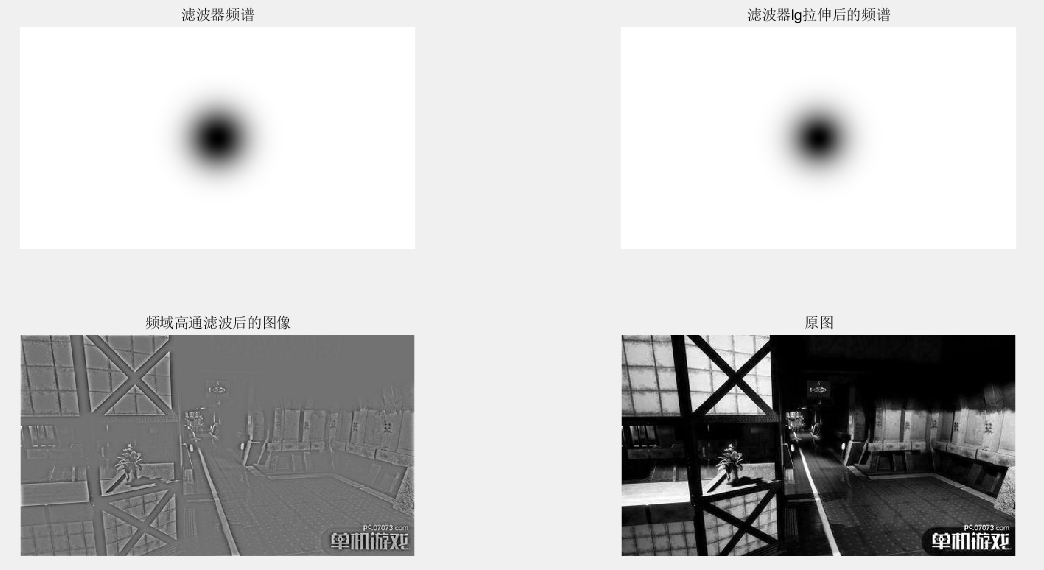%锐化频域滤波器
%若有低通的传递函数H（u，v）  高通滤波器可由下式得出
%H（u,v）=1-Hlp(u,v)
%这副图像的边缘和其他亮度得到了变化  由于图像的平均值由 0，0给处
%而且至今为止，高通滤波器偏离了傅里叶变换的原点  图像失去了大部分原图像所呈现的背景色彩
%这个问题在高频强调滤波器中讲明（下一个博客）
f=f(:,:,1);
PQ=[size(f,1)*2,size(f,2)*2];
[U,V]=dftuv(PQ(1),PQ(2));
D0=0.05*PQ(2);%使用D0的值等于填充后图像宽度的5% 当D=D0时 截至频率
F=fft2(f,PQ(1),PQ(2));
H=exp(-(U.^2+V.^2)/(2*(D0^2)));%高斯低通滤波器
H=1-H;
g=dftfilt(f,H);
subplot(2,2,1)
imshow(fftshift(H),[])
title('滤波器频谱')

subplot(2,2,2)
imshow(log(1+abs(fftshift(H))),[])
title('滤波器lg拉伸后的频谱')

subplot(2,2,3)
imshow(g,[])
title('频域高通滤波后的图像')

subplot(2,2,4)
imshow(f,[])
title('原图')

2017-12-03 17:28:28 arryCC 阅读数 3662
• ###### MATLAB图像处理

全面系统的学习MATLAB在图像处理中的应用

20788 人正在学习 去看看 魏伟

# 数字图像处理

## 一、学习内容总结

### 1. 第一章 绪论

1. 定义我们称之为数字图像处理领域的范围；
2. 通过考察几个领域，给出图像处理技术状况的概念；
3. 讨论图像处理用到的几种方法；
4. 概述通用目的的典型图像处理系统的组成。

#### 1.1 什么是数字图像处理

• 强度灰度：一幅图像可以被定义为一个二维函数 $f\left(x,y\right)$$f(x,y)$，其中 $x,y$$x,y$ 是空间（平面）坐标，而在任何一处的幅值 $f$$f$ 被称为在该点的灰度或强度。
• 数字图像：当 $x,y$$x,y$ 或灰度值 $f$$f$有限的离散数值时，称该图像为数字图像。也就是说数字图像是由有限数量的元素组成，每个元素都有特定的位置和幅值。这些元素被称为图画元素图像元素像素
• 数字图像处理 : 指用特定的计算机来处理数字图像。

#### 1.2 使用数字图像处理领域的实例

• 伽马射线成像：医学和天文。
• X射线成像：最早用于成像的电磁辐射源之一，医学诊断。
• 紫外波段成像 ：荧光显微镜。
• 可见光及红外线成像 ：可见显微镜技术，遥感，天气预测和预报，红外卫星图像，自动视觉检测，检测丢失的部件，指纹图像。
• 微波波段成像 ：雷达。
• 无线电波段成像 ：天文学和医学（核磁共振）。
• 其他方式 ：声波成像，电子显微镜方法，（由计算机产生的）合成图像。

#### 1.3 数字图像处理的基本步骤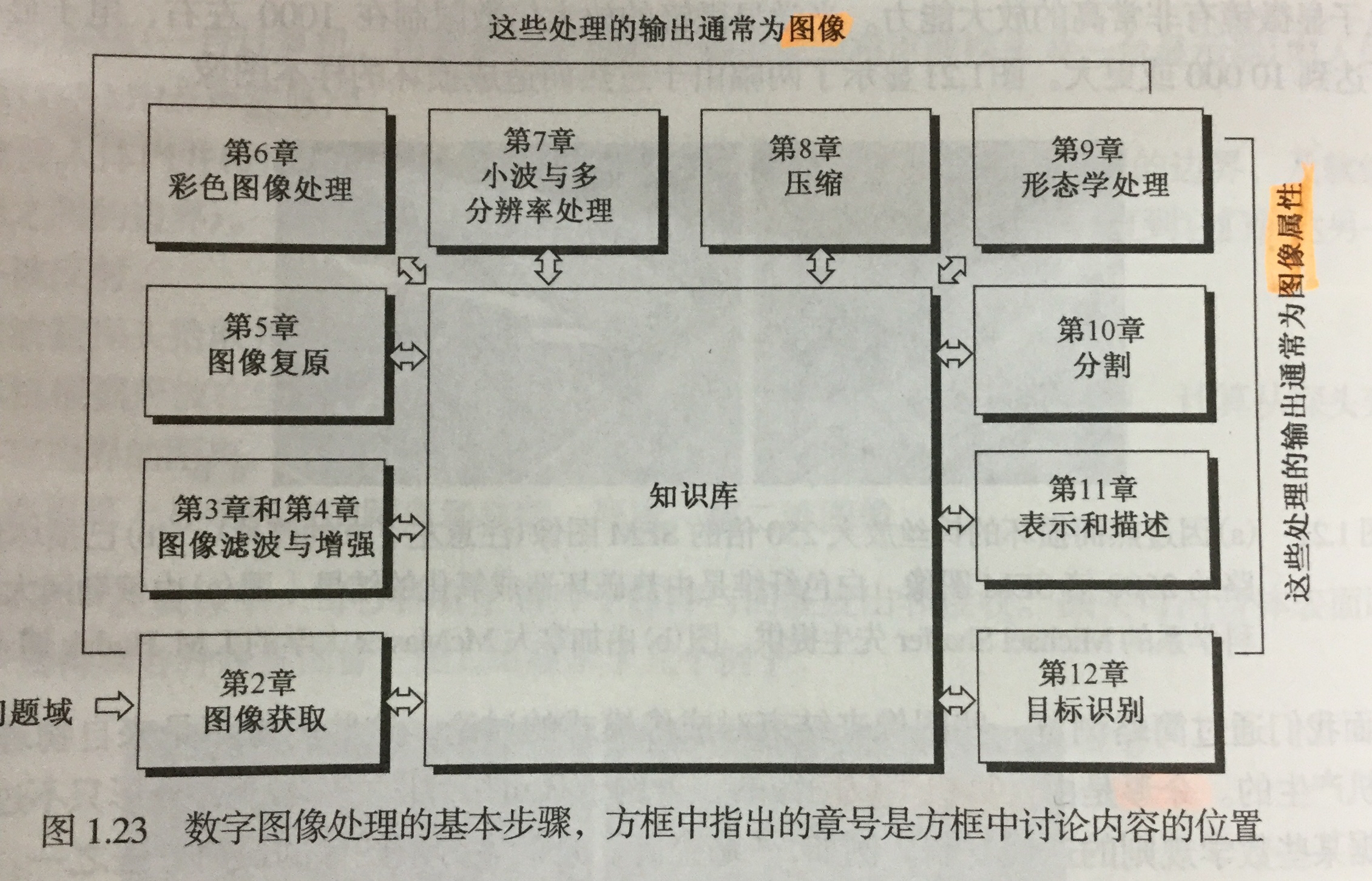#### 1.4 图像处理系统的组成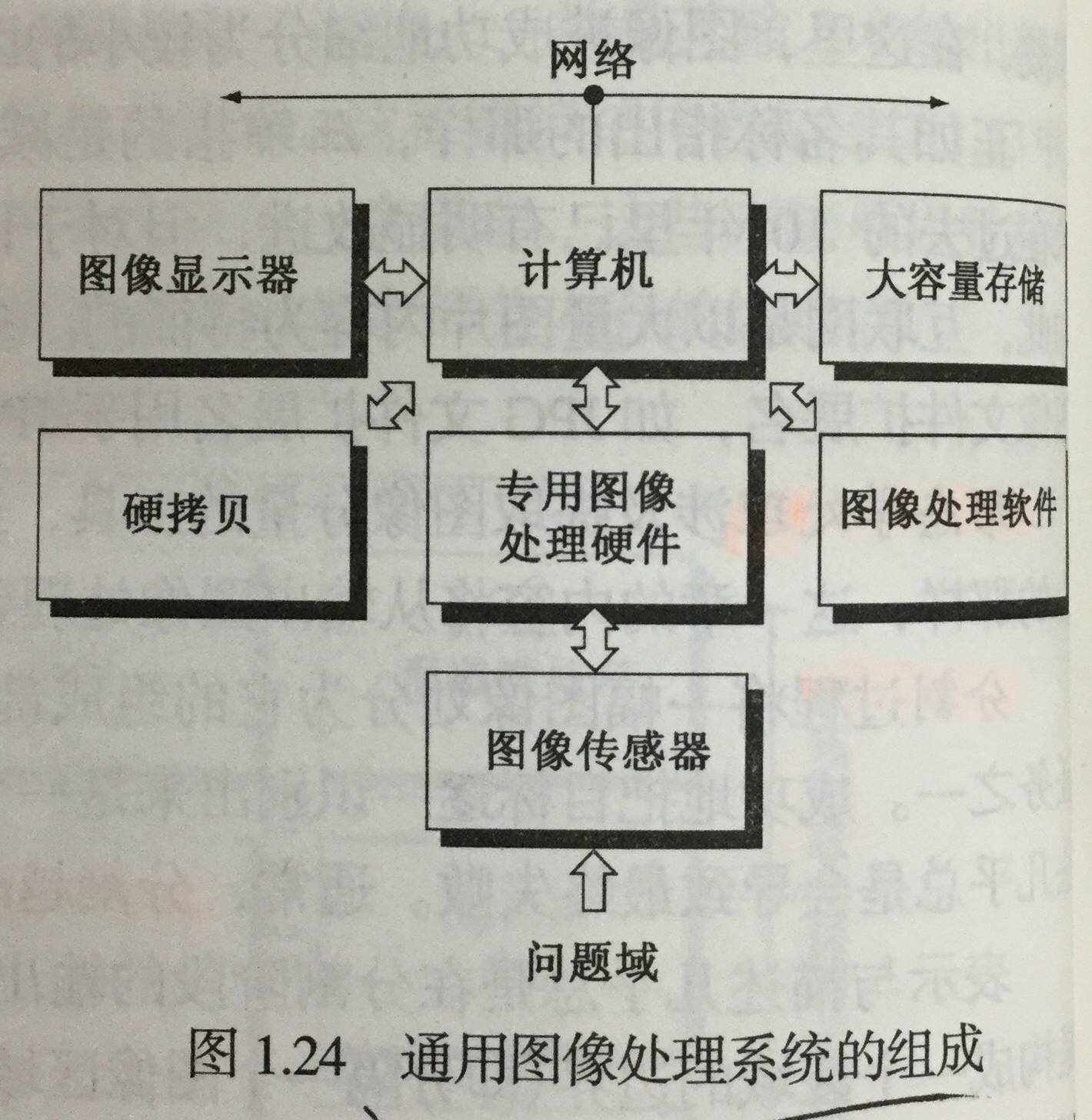### 2. 第二章 数字图像处理基础

#### 2.1 视觉感知要素

• 人眼的结构

重点介绍视网膜里的两类光感受器

• 锥状体 ：对颜色高度敏感，这种视觉称为白昼视觉或者亮视觉。高照明水平下执行。
• 杆状体 ：没有彩色感觉，对低照明度敏感，称为暗视觉或微光视觉。低照明水平下执行。
• 亮度适应与辨别

• 亮度适应现象 ：视觉系统不能同时在一个范围内工作，它是通过改变其整个灵敏度来完成这一较大变动的。
• 韦伯比 ：较大：亮度辨别能力较差；反之，较好。
• 感知亮度 不是简单的强度的函数

• 视觉系统往往会在不同强度区域的边界处出现“下冲”或“上冲”现象。
• 同时对比、错觉

#### 2.2 光和电磁波谱

• 电磁波是能量的一种，任何有能量的物体都会释放电磁波谱。它可以用 波长 ($\lambda$$\lambda$)、频率($v$$v$)或能量($E$$E$) 来描述，其中

$\lambda =c/v$

$E=hv$

• 光是一种特殊的电磁辐射，可以被人眼感知。
• 单色光 是没有颜色的光，也成为无色光。唯一属性就是它的强度或者大小，用 灰度级 来表示。单色图像常被称为 灰度图像
• 彩色光源 的质量可以用发光强度、光通量和亮度 来表示。

#### 2.3 图像感知和获取

• 图像获取方式

• 使用单个传感器来获取图像
• 使用条带传感器获取图像
• 使用传感器阵列获取图像
• 简单的图像形成模型

用形如 $f\left(x,y\right)$$f(x,y)$ 的二维函数来表示图像，那么：

$0

$f\left(x,y\right)$$f(x,y)$ 可以用两个分量来表征：

• 入射分量 入射到被观察场景的光源照射总量，用$i\left(x,y\right)$$i(x,y)$ 表示;
• 反射分量 场景中物体所反射的光照总量，用$r\left(x,y\right)$$r(x,y)$ 表示。

所以有：

$f\left(x,y\right)=i\left(x,y\right)r\left(x,y\right),0

#### 2.4 图像取样和量化

• 取样和量化的基本概念

• 取样 ：对坐标值进行数字化
• 量化 ： 对幅值数字化

数字图像的质量在很大程度上取决于取样和量化中所用的样本数灰度级

• 数字图像表示

用数列矩阵来表示一幅数字图像。在实数矩阵中，每个元素称为图像单元、图像元素或像素。

对比度 一幅图像最高和最低灰度级间的灰度差为对比度。

存储数字图像所用的比特数为：

$b=M×N×k,当M=N时，b={N}^{2}k$

灰度级数$L={2}^{k}$$L = 2^k$

• 空间和灰度分辨率

• 空间分辨率 ：图像中可辨别的最小细节的度量。在数量上，表示每单位距离线对数和每单位距离点数是最通用的度量（必须针对空间单位来规定才有意义）。
• 灰度分辨率 ：指在灰度级中可分辨的最小变化。
• 图像内插

用已知数据来估计未知位置的数据处理。是基本的图像重取样方法。可以处理图像的放大和缩小。

#### 2.5 像素间的基本关系

• 相邻像素

位于坐标 $\left(x,y\right)$$(x,y)$ 处的像素 $p$$p$ 有4个水平和垂直上的相邻像素，用 ${N}_{4}\left(p\right)$$N_4(p)$ 表示；有四个对角相邻像素，用 ${N}_{D}\left(p\right)$$N_D(p)$ 表示。如果 $p$$p$ 位于图像边界，则某些邻点可能 落在图像外边。

• 邻接性、连通性、区域和边界

• 4邻接、8邻接、混合邻接
• 距离度量

• 欧氏距离(圆)
• ${D}_{4}$$D_4$ 城市街区距离(菱形)
• 棋盘距离(正方形)

#### 2.6 常用数学工具介绍

• 阵列和矩阵操作
• 线性操作和非线性操作
• 算术操作
• 集合和逻辑操作
• 基本集合操作
• 逻辑操作
• 模糊集合
• 空间操作
• 单像素操作
• 邻域操作
• 几何空间变换与图像配准
• 向量和矩阵操作
• 图像变换

### 3.第三章

#### 3.1 背景知识

• 空间域 就是简单的包含图像像素的平面。空间域处理可用以下方式表示：

$g\left(x,y\right)=T\left[f\left(x,y\right)\right],T是在点\left(x,y\right)的邻域上处定义的一种算子$

• 灰度变换函数
$s=T\left(r\right),r,s分别代表处理前后的像素值$

#### 3.2 基本灰度变换函数

• 图像反转

得到灰度范围为 $\left[0,L-1\right]$$[0,L-1]$ 的一幅图像的反转图像:（得到等效的照片底片）

$s=L-1-r$

• 对数变换

对数变换的通用形式：

$s=clog\left(1+r\right)$

扩展图像中暗像素的值，同时压缩更高灰度级的值。反对数变换的作用与此相反。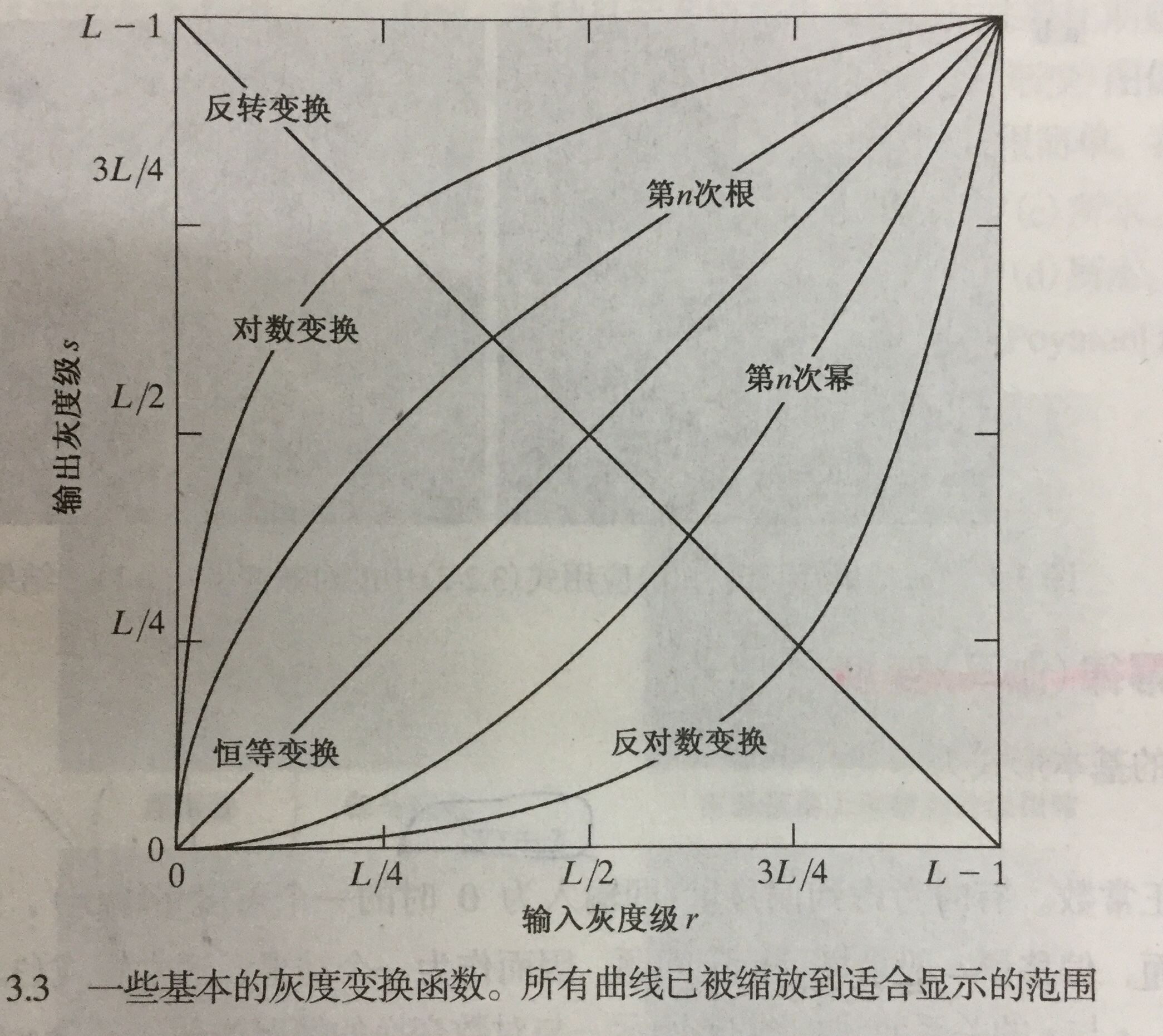• 幂律变换(伽马)变换

基本形式：

$s=c{r}^{\gamma }$

$\gamma <1$$\gamma < 1$ 变亮，大于1变暗，$c=\gamma =1$$c = \gamma = 1$ 恒等变换。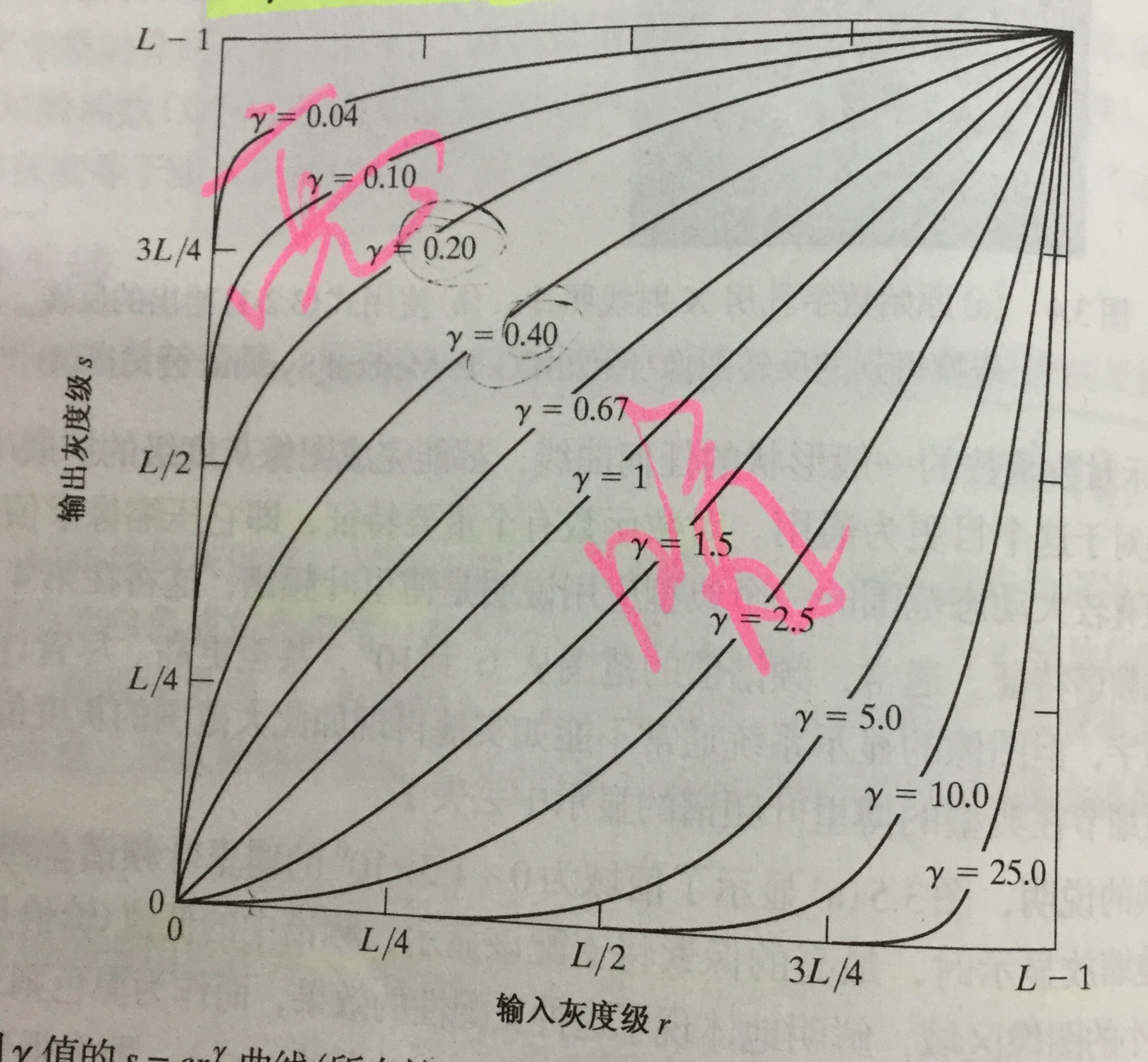* 分段线性变换函数
* 对比度拉伸：扩展图像灰度级动态范围处理，因此它可以跨越记录介质和显示装置的全部灰度范围。

根据$r,s$ 的取值，变换可以为线性函数和阈值处理函数。

• 灰度级分层：突出特定图像灰度范围的亮度。有两种方法：

• 突出范围 $\left[A,B\right]$$[A,B]$ 内的灰度，并将所有其他灰度降低到一个更低的级别；
• 突出范围$\left[A,N\right]$$[A,N]$ 内的灰度，并保持所有其他灰度级不变。
• 比特平面分层：突出特定比特为整个图像外观作贡献。

• 4个高阶比特平面，特别是最后两个比特平面，包含了在视觉上很重要的大多数数据。
• 低阶比特平面在图像中贡献更精细的灰度细节。

得出结论：储存四个高阶比特平面将允许我们以可接受的细节来重建原图像。这样可减少50%的存储量。

#### 3.3 直方图的处理

• 理论基础：若一幅图像的像素倾向于占据可能的灰度级并且分布均匀，则该图像会有高对比度的外观并展示灰色调的较大变化。

• 直方图均衡：

• 灰度范围为 $\left[0,L-1\right]$$[0,L-1]$ 的数字图像的直方图是离散函数 $h\left({r}_{k}\right)={n}_{k}$$h(r_k) = n_k$,其中 ${r}_{k}$$r_k$ 是第 $k$$k$ 级灰度值，${n}_{k}$$n_k$ 是图像中灰度为${r}_{k}$$r_k$ 的像素的个数。
• 通过转换函数$T\left({r}_{k}\right)$$T(r_k)$变换，得到直方图均衡化。
• 应用：自适应对比度增强。
• 直方图匹配：用于处理后有特殊直方图的方法。

• 局部直方图处理：以图像中每个像素邻域中的灰度分布为基础设计变换函数，来增强图像中小区域的细节。

• 在图像增强中使用直方图统计：提供这样一种增强图像的方法：

在仅处理均值和方差时，实际上直接从取样值来估计它们，不必计算直方图。这些估计被称为取样均值和取样方差。

#### 3.4 空间滤波基础

• 空间滤波机理

• 空间滤波器的组成：
• 一个邻域
• 对该邻域包围的图像像素执行的预定义操作

滤波产生的是一个新像素，新像素的坐标等于邻域中心的坐标，像素的值是滤波操作的结果。

• 空间相关与卷积

• 相关：滤波器模板移过图像并计算每个位置乘积之和的处理。一个大小为$m×n$$m×n$ 的滤波器与一幅图像 $f\left(x,y\right)$$f(x,y)$ 做相关操作，可表示为$w\left(x,y\right)☆f\left(x,y\right)$$w(x,y)☆f(x,y)$
• 卷积：与相关机理相似，但滤波器首先要旋转${180}^{o}$$180^o$ 一个大小为$m×n$$m×n$ 的滤波器与一幅图像 $f\left(x,y\right)$$f(x,y)$ 做j卷积操作，可表示为$w\left(x,y\right)★f\left(x,y\right)$$w(x,y)★f(x,y)$

#### 3.5 平滑空间滤波器

• 平滑线性滤波器(均值滤波器)

它使用滤波器确定的邻域内像素的平均灰度值代替图像中每个像素的值。应用：

• 降低噪声
• 灰度级数量不足而引起的伪轮廓效应的平滑处理
• 去除图像的不相关细节
• 统计排序(非线性)滤波器

最有代表性的是中值滤波器 ，特点：

• 将像素邻域内灰度的中值(在中值计算中，包括原像素值)代替该像素的值；
• 对处理脉冲噪声(椒盐噪声)非常有效。

#### 3.6 锐化空间滤波器

• 拉普拉斯算子：最简单的各向同性微分算子，是一个线性算子。因其为微分算子，因此强调的是图像中灰度的 突变而不是灰度级缓慢变换的区域。

• 非锐化隐蔽和高提升滤波：从原图像中减去一部分非锐化的版本。步骤：

• 模糊原图像
• 从原图像减去模糊图像
• 将模板加到原图像上
• 梯度：图像处理中的一阶微分用梯度实现。对于函数$f\left(x\right)$$f(x)$ ，在坐标$\left(x,y\right)$$(x,y)$ 处的梯度定义为二维列向量。它指出在位置$f\left(x,y\right)$$f(x,y)$$f$$f$的最大变化率方向。

应用：边缘增强。

### 4.第四章

#### 4.1. 基本概念

• 傅里叶概念：任何周期函数都可以表示为不同频率的正弦和或余弦和的形式，每个正弦项和或余弦项乘以不同的系数（傅里叶级数）。
• 傅里叶变换：在非周期函数用正弦和或余弦和乘以加权函数的积分来表示的公式。
• 介绍复数、傅里叶级数、冲击及其取样特征、连续函数的傅里叶变换以及之前提过的卷积。

#### 4.2. 取样与取样函数中的傅里叶变换

• 取样

在连续函数$f\left(x,y\right)$$f(x,y)$ 中模拟取样的一种方法是：用一个$\mathrm{\Delta }T$$\Delta T$ 单位间隔的冲击串作为取样函数去乘以$f\left(t\right)$$f(t)$ .

• 取样函数的傅里叶变换

空间域来两个函数乘积的傅里叶变换是两个函数在频率域的卷积。

• 取样定理

如果以超过函数最高频率的两倍的取样来获取样本，连续的带限函数可以完全从它的样本集来恢复。

#### 4.3. DFT小结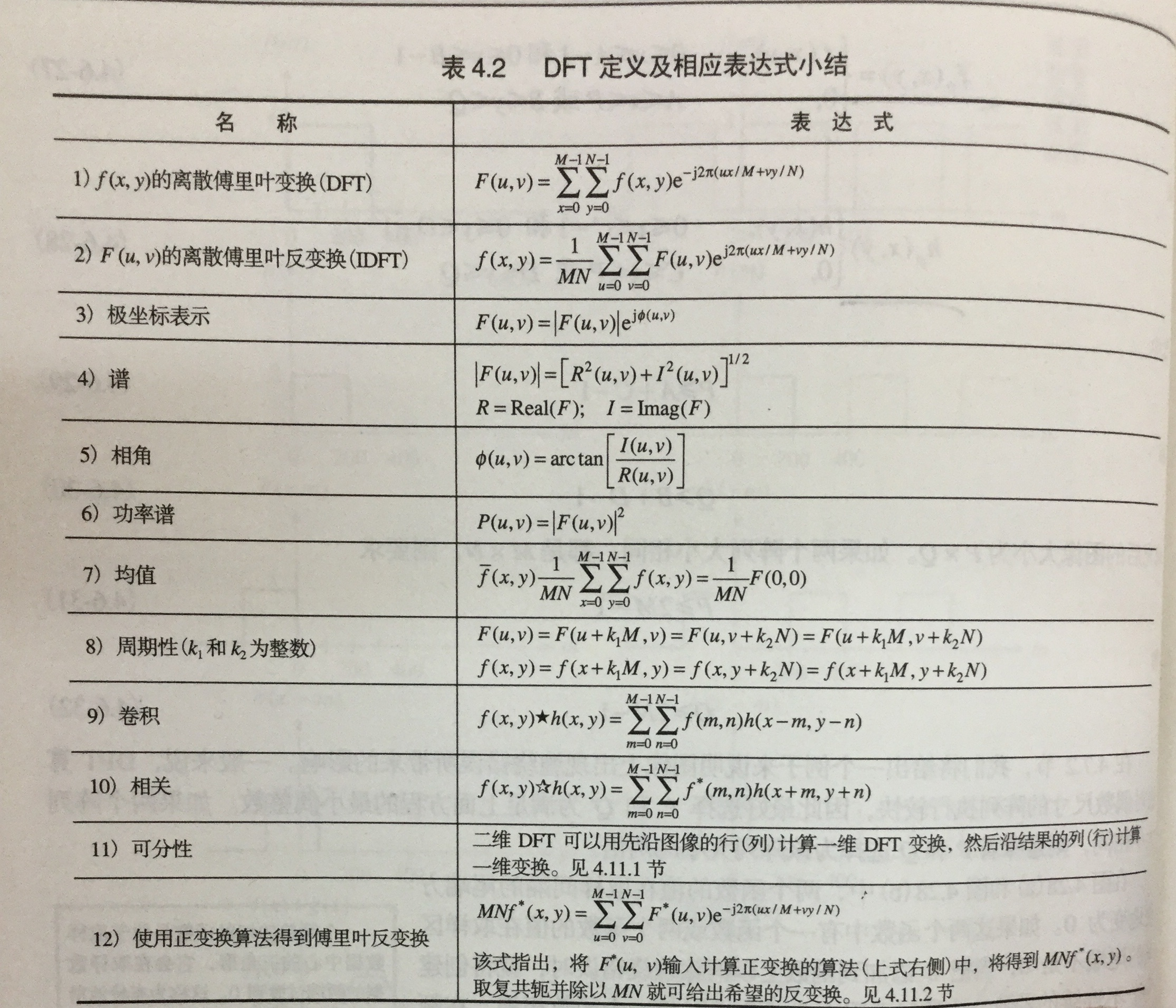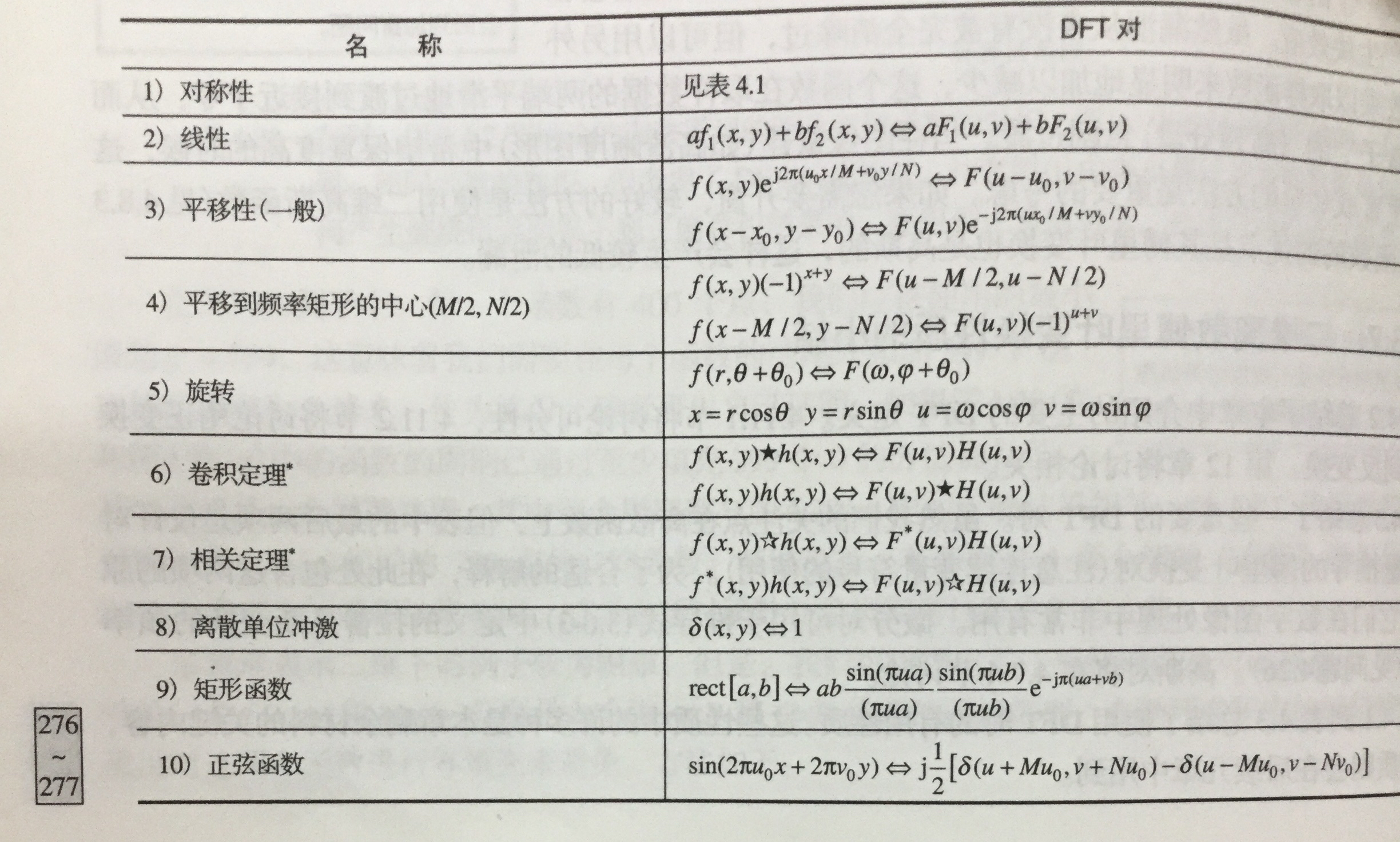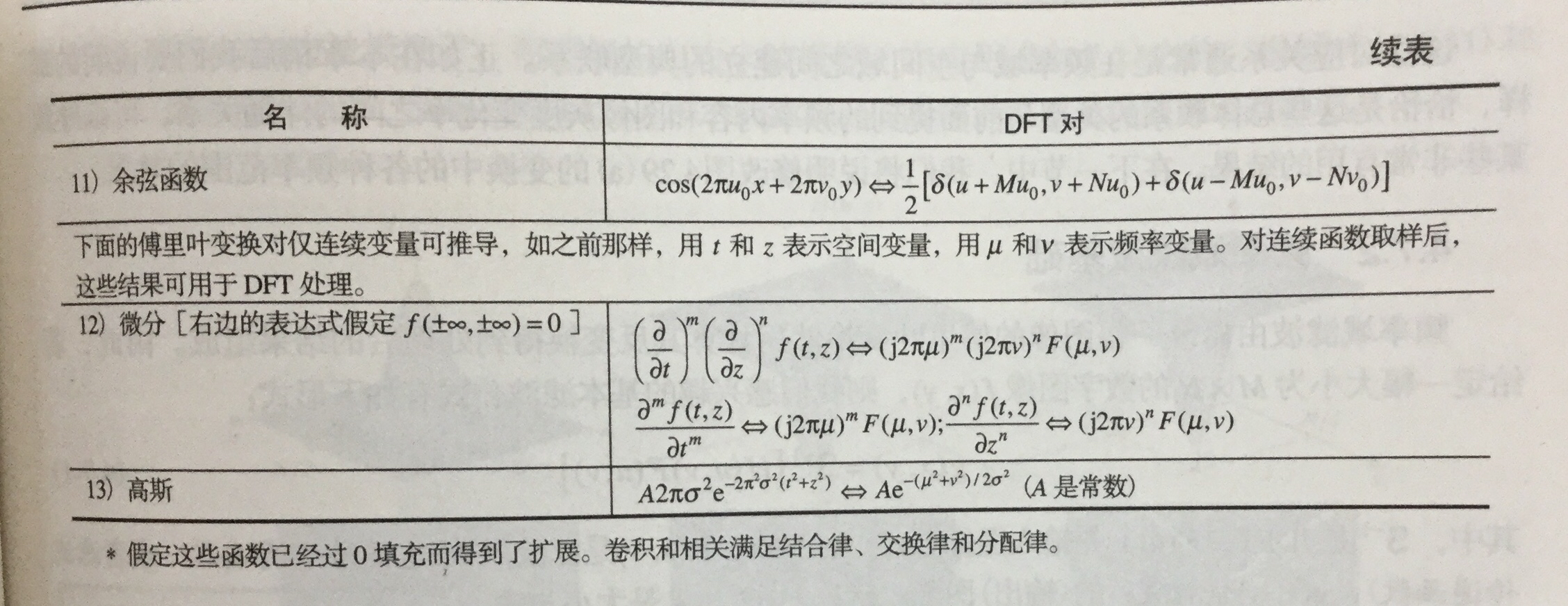#### 4.4. 频率域滤波

• 步骤
• 等到填充参数$P$$P$$Q$$Q$
• 形成大小为$P×Q$$P × Q$ 的填充后的图像${f}_{p}\left(x,y\right)$$f_p(x,y)$
• $\left(-1{\right)}^{x+y}$$(-1)^{x+y}$ 乘以${f}_{p}\left(x,y\right)$$f_p(x,y)$移到其变换中心
• 计算上一步骤的DTF，得到$F\left(u,v\right)$$F(u,v)$
• 生成实的、对称的滤波函数$H\left(u,v\right)$$H(u,v)$
• 得到处理后的图像${g}_{p}\left(x,y\right)$$g_p(x,y)$
• ${g}_{p}\left(x,y\right)$$g_p(x,y)$ 的做上限提取$M×N$$M×N$区域 ，得到最终的处理结果$g\left(x,y\right)$$g(x,y)$
• 空间域与频率域间的纽带是卷积定理。

#### 4.5. 使用频率域滤波器平滑图像

• 定义总结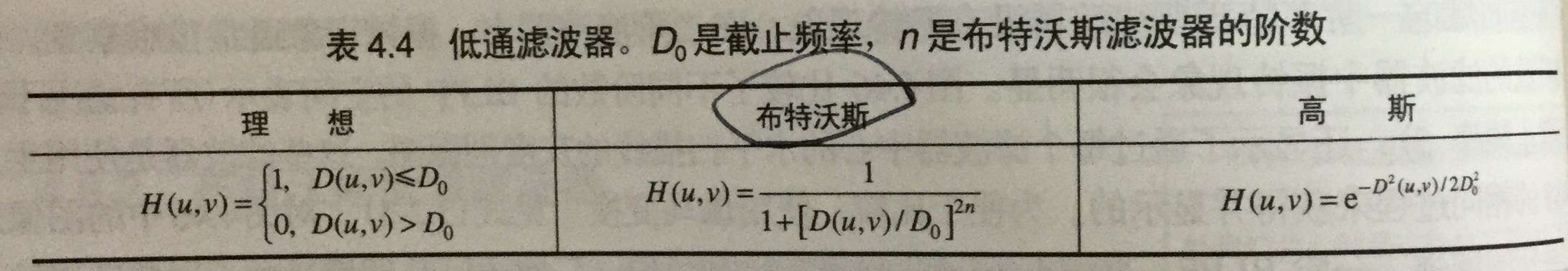• 特性
• 理想低通滤波器(ILFP)
• 特性：模糊和振铃。
• 布特沃斯低通滤波器(BLPF)
• 特性：随着阶数增高，其振铃和负值变明显。(一阶时无)
• 高斯低通滤波器(GLPF)
• 特性：无振铃

#### 4.6. 使用频率域滤波器锐化图像

• 三种高通滤波器来锐化图像
• 定义总结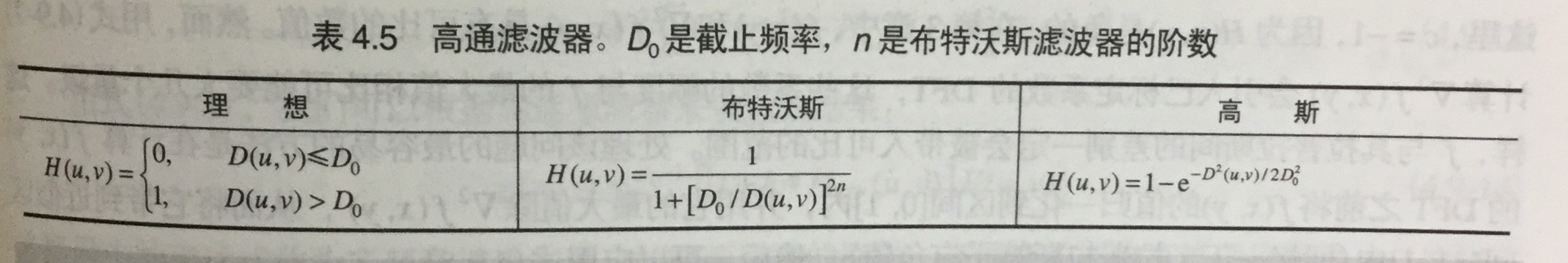* 特性

* 理想高通滤波器(IHPF)
* 有振铃
* 布特沃斯高通滤波器(BHPF)
* 比IHPF更平滑
* 高斯低通滤波器(GHPF)
* 比前两个更平滑，即使微小物体和细线条得到的结果也比较其清晰

• 其他方式

• 拉普拉斯算子
• 钝化模板、高提升滤波和高频强调滤波
• 同态滤波

#### 4.7.选择性滤波器

• 带阻滤波器和带通滤波器

• 带阻滤波器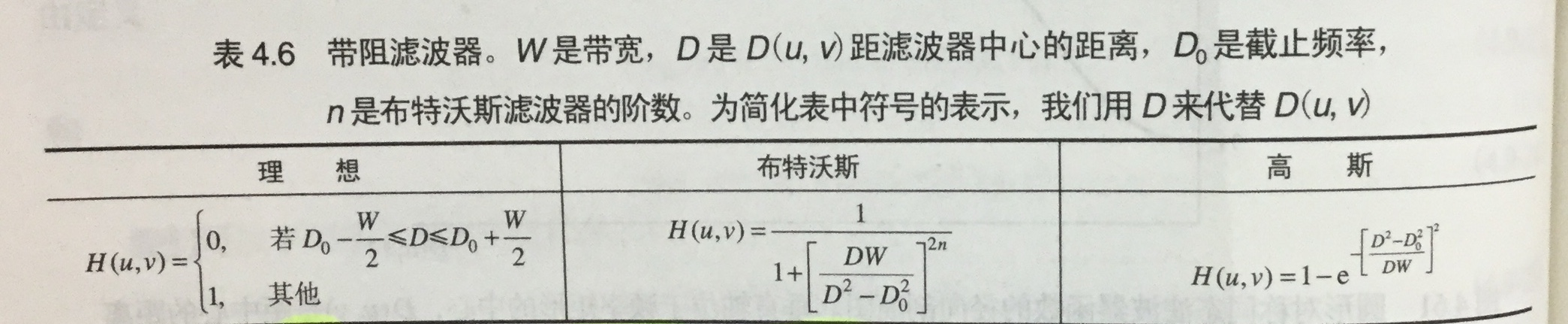• 带通滤波器

• 通过1减去带阻得到。

• 陷波滤波器：拒绝事先定义的关于频率矩形中心的一个邻域的频率。

• 陷波带阻滤波器

用中心已被平移到陷波滤波中心的高通滤波器的乘积来构造。

• 陷波带通滤波器

通过1减去带阻得到。

2018-01-04 14:08:25 baidu_34971492 阅读数 41029
• ###### MATLAB图像处理

全面系统的学习MATLAB在图像处理中的应用

20788 人正在学习 去看看 魏伟

1.  背景知识

 2 4 8 1 3 9 5 7 6

g（x,y）=med{f(x-k,y-l),(k,l∈W)}

g =med[2,4,8;1,3,9;5,7,6] = 5

 5

 2 4 8 1 3 9 5 7 6

gxy=1/m ∑fxy

g = (1/8)*(2+4+8+1+9+5+7+6)= 5

 5

2.  MATLAB实现

%%-------------------------------------------------------------------------------------------

%% 2018/01/03

%% lee

%% 137194782@qq.com

%% 微信公众号：FPGA开源工作室

%%--------------------------------------------------------------------------------------------

clear all;

clc;

figure,imshow(M);                %显示原始图像

title('original');

gray = rgb2gray(M);

figure,imshow(gray);                         %显示灰度图像

title('gray');

P1 = imnoise(gray,'gaussian',0.02);     %加入高斯躁声

figure,imshow(P1);                        %加入高斯躁声后显示图像

title('gaussiannoise');

P2 = imnoise(gray,'salt& pepper',0.02); %加入椒盐躁声

figure,imshow(P2);                        %加入椒盐躁声后显示图像

title('salt& pepper noise');

g = medfilt2(P1);                       %对高斯躁声中值滤波

figure,imshow(g);

title('medfiltergaussian');

h = medfilt2(P2);                       %对椒盐躁声中值滤波

figure,imshow(h);

title('medfiltersalt & pepper noise');

a=[1 1 1                               %对高斯躁声算术均值滤波

1 11

1 11];

l=1/9*a;

k = conv2(double(P1),double(l));

figure,imshow(k,[]);

title('arithmeticfiltergaussian');

d = conv2(double(P2),double(l));           %对椒盐躁声算术均值滤波

figure,imshow(d,[]);

title('arithmeticfiltersalt & pepper noise');

sigma=8;%标准差大小

window=double(uint8(3*sigma)*2+1);%窗口大小一半为3*sigma

H=fspecial('gaussian',window, sigma);%fspecial('gaussian',hsize, sigma)产生滤波模板

img_gauss=imfilter(P1,H,'replicate'); %为了不出现黑边，使用参数'replicate'（输入图像的外部边界通过复制内部边界的值来扩展）

figure, imshow(img_gauss);

title('gaussianfilting gauss noise');

img_salt=imfilter(P2,H,'replicate');

figure, imshow(img_salt);

title('gaussianfilting salt pepper noise');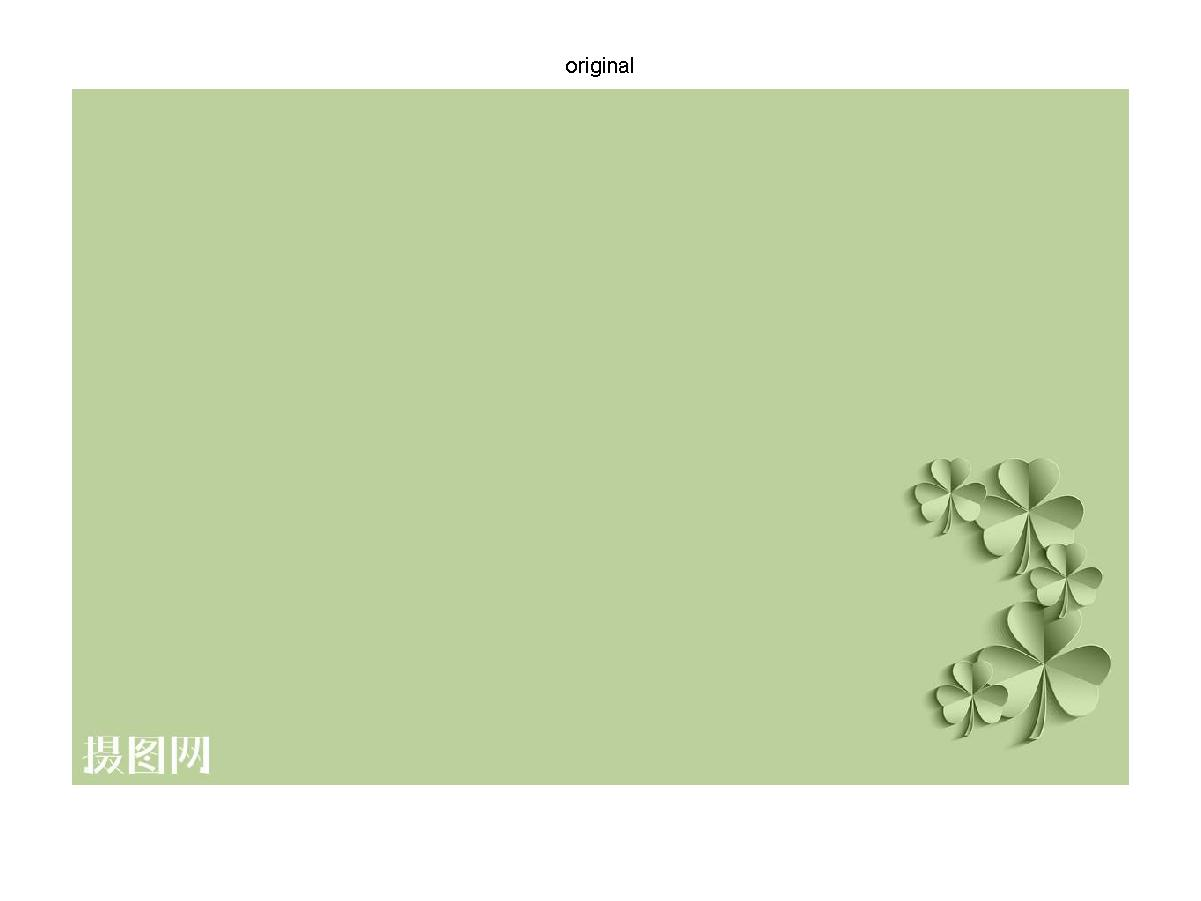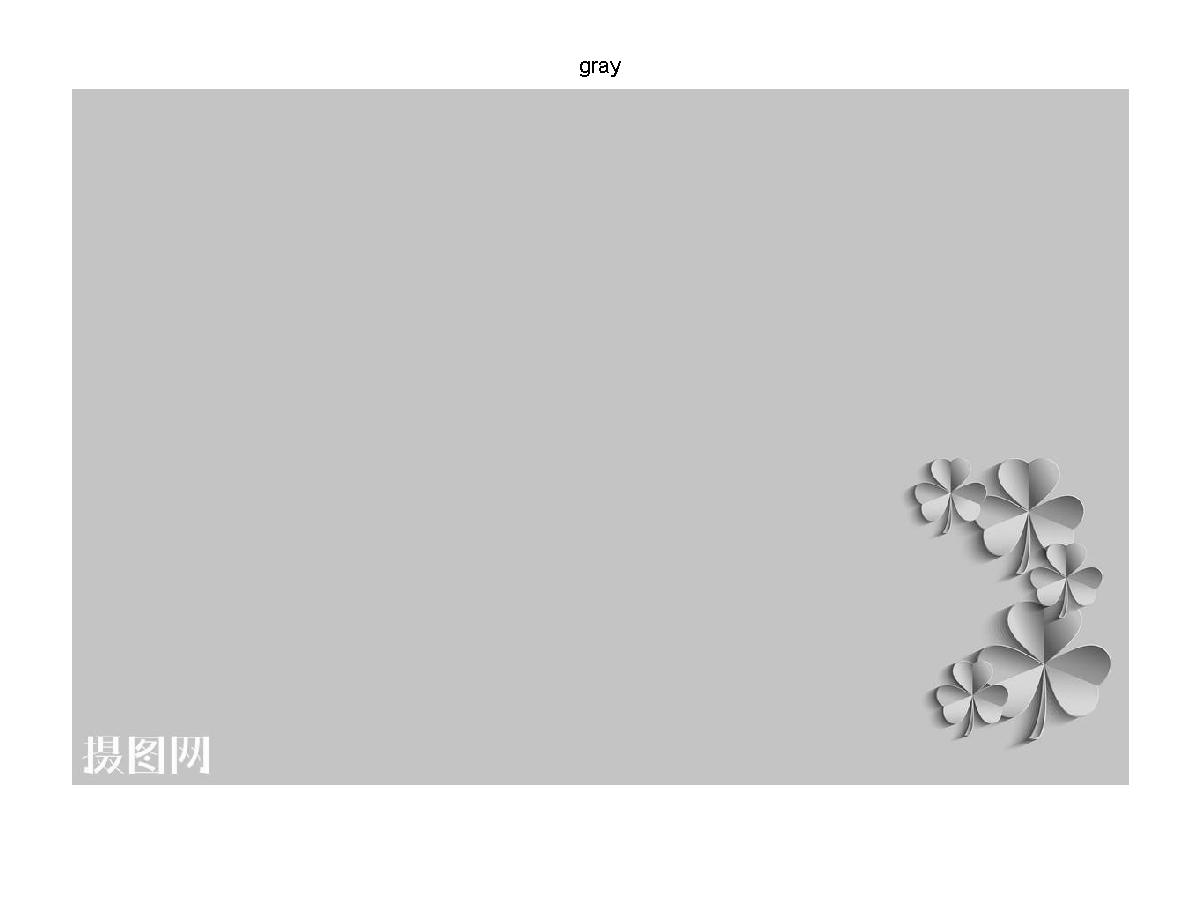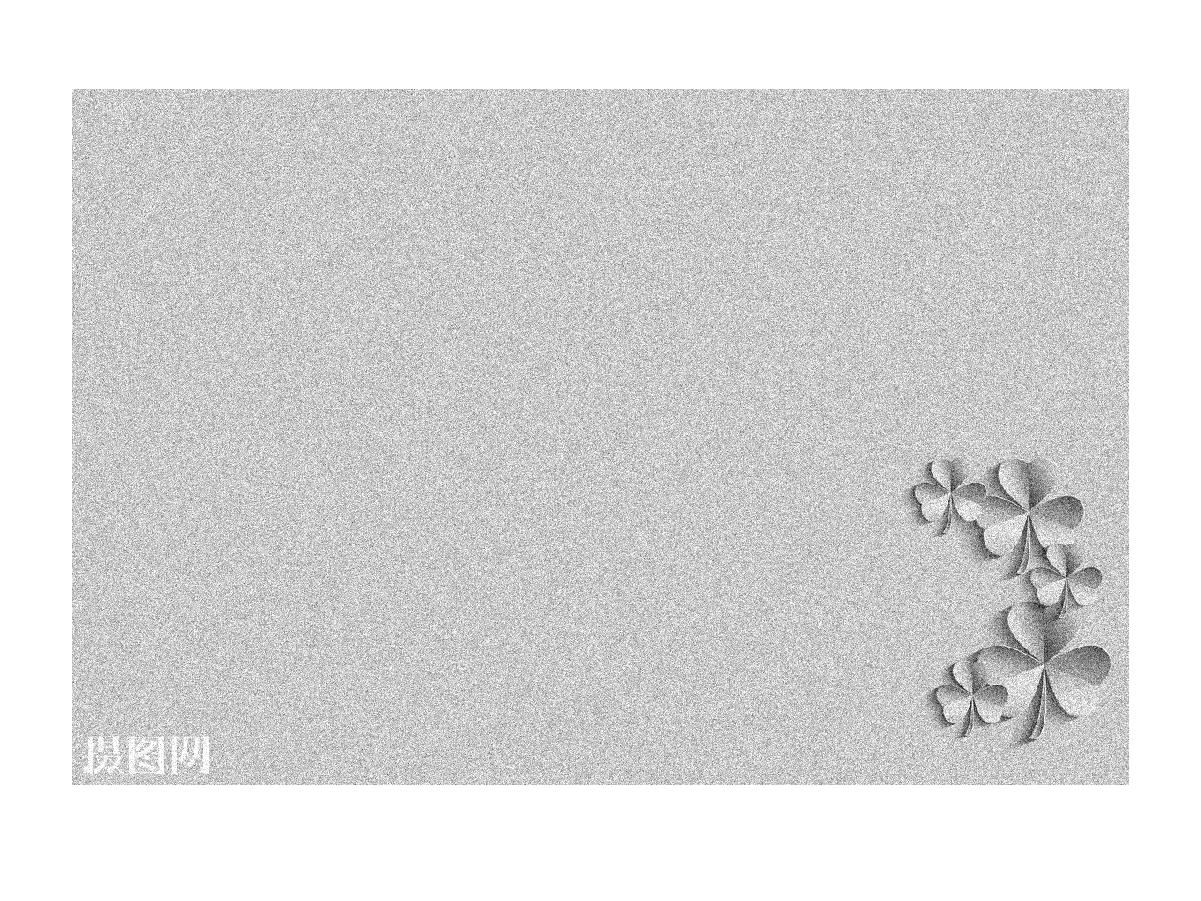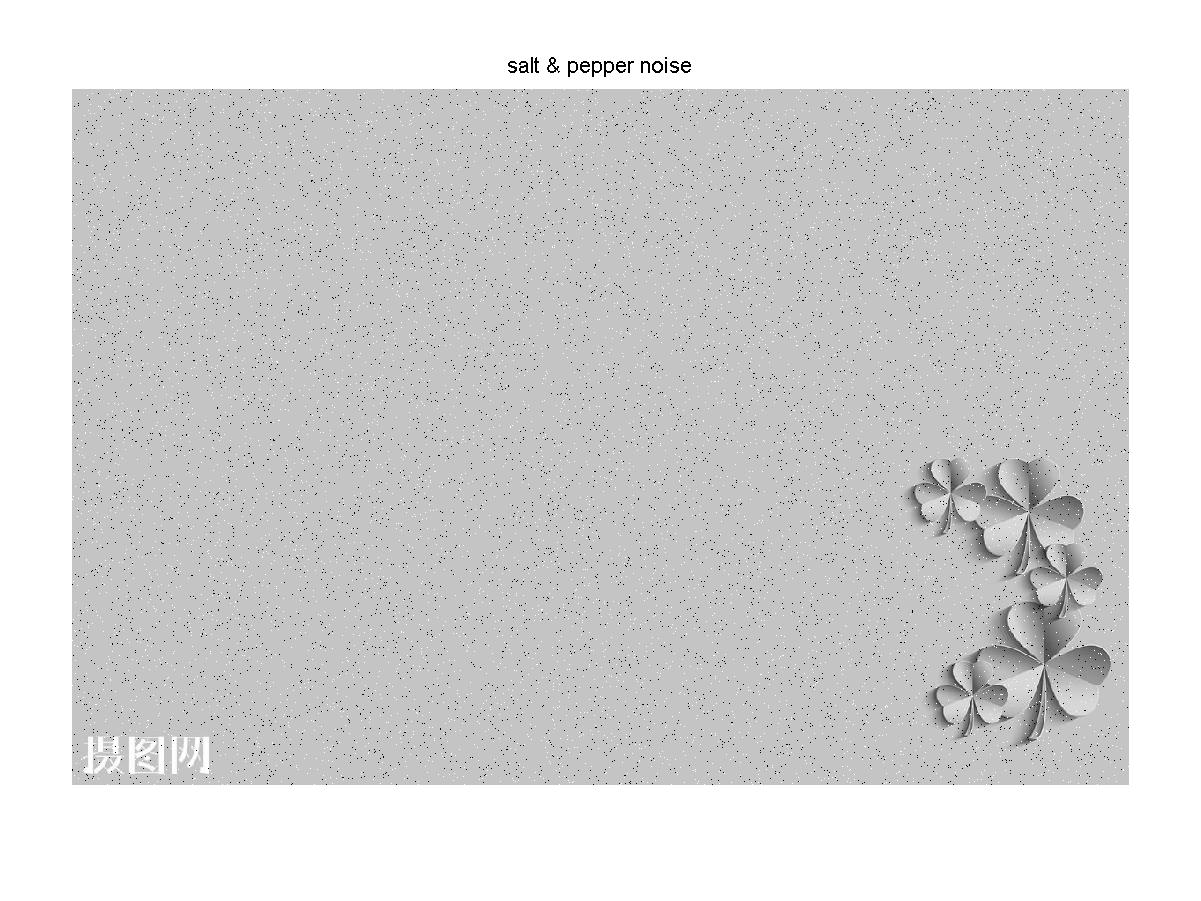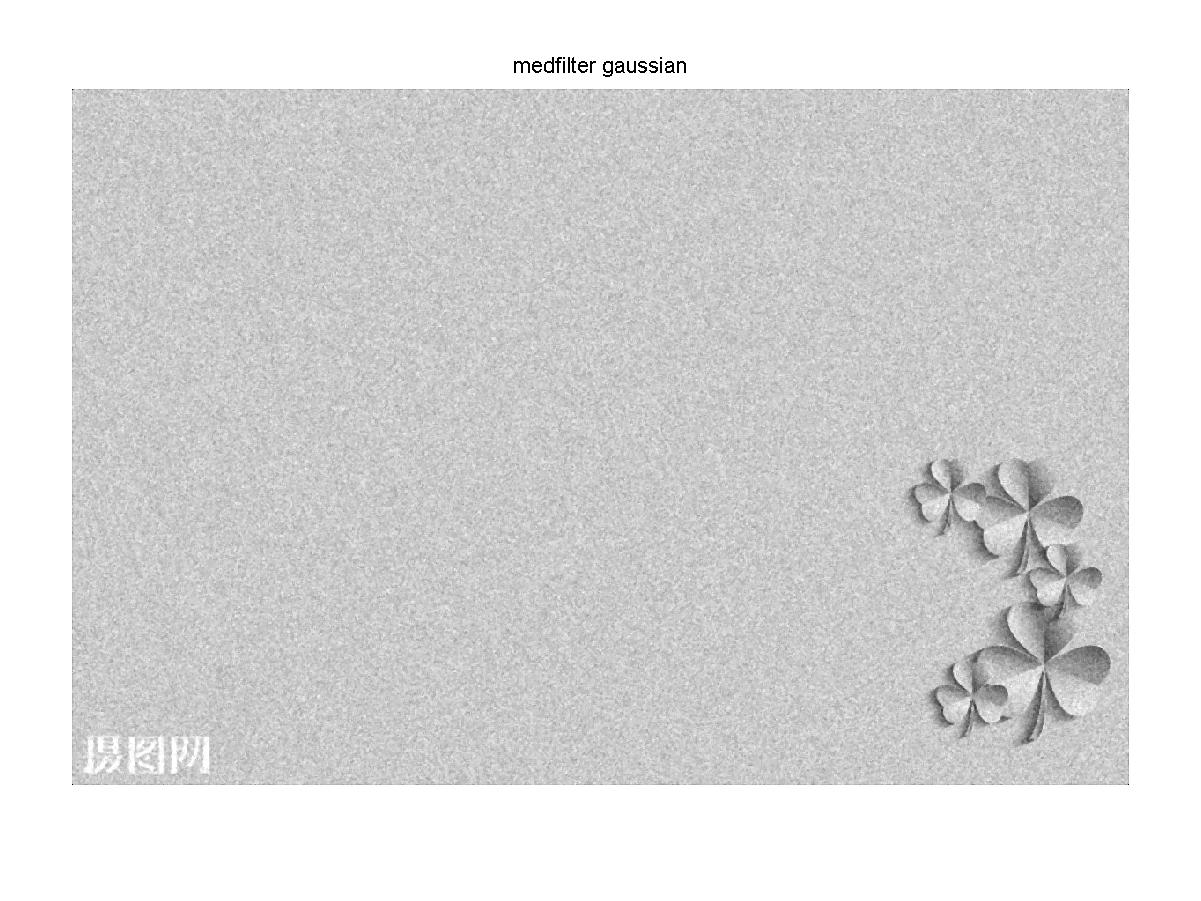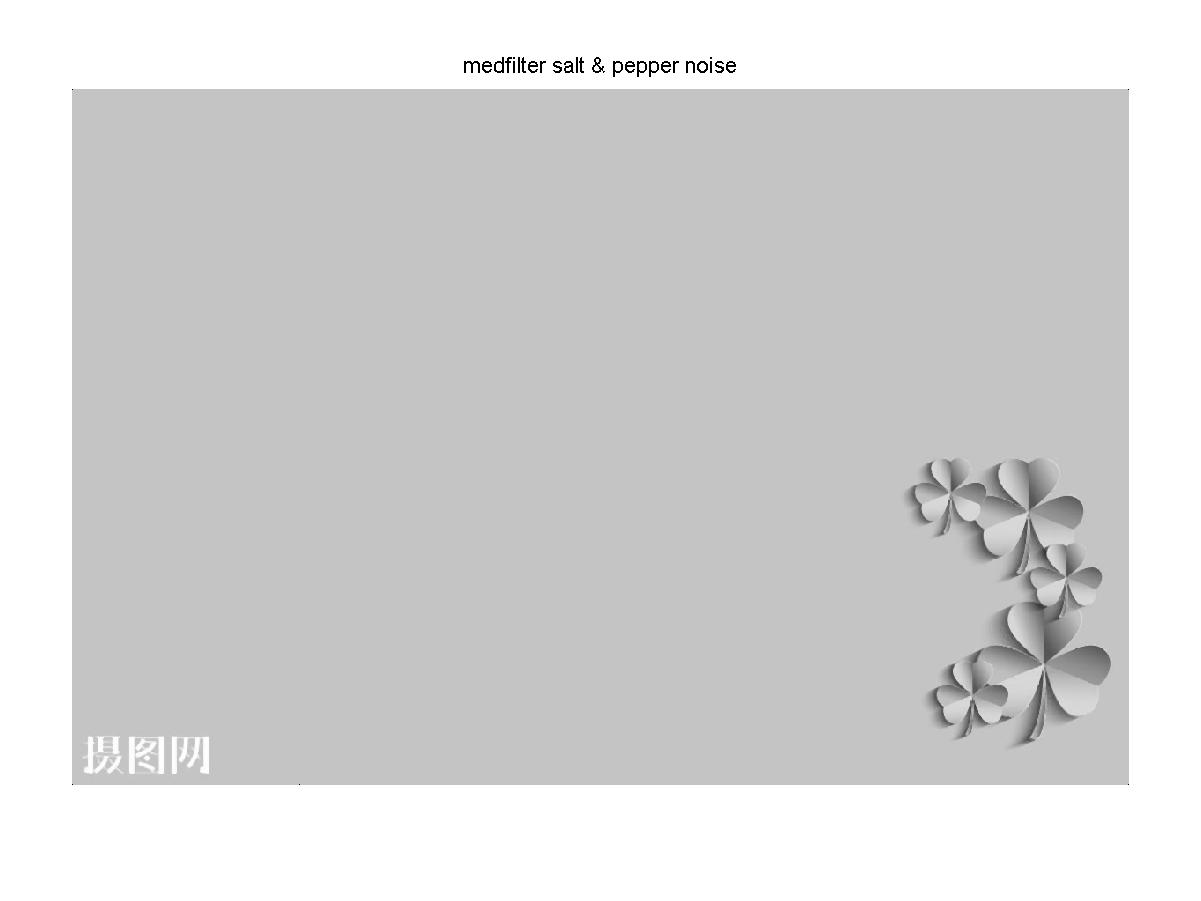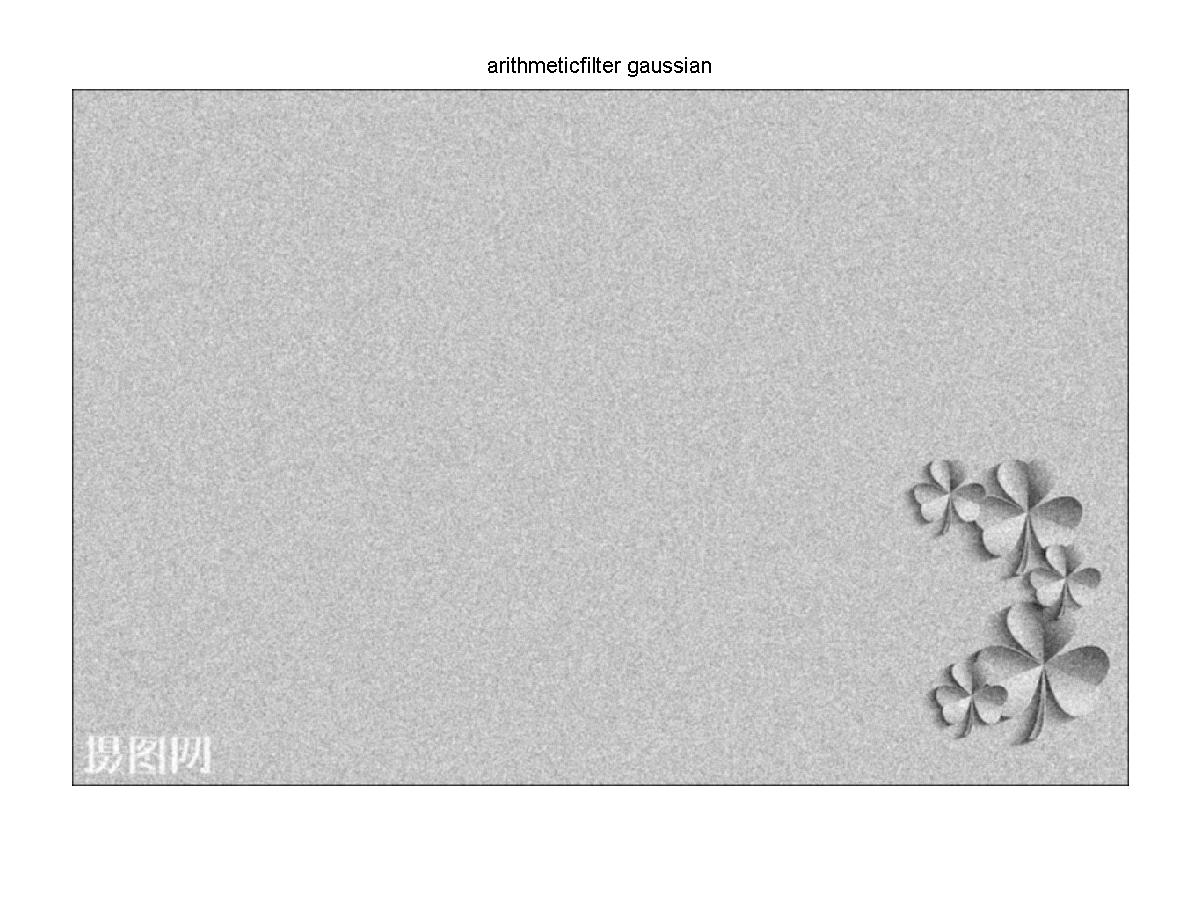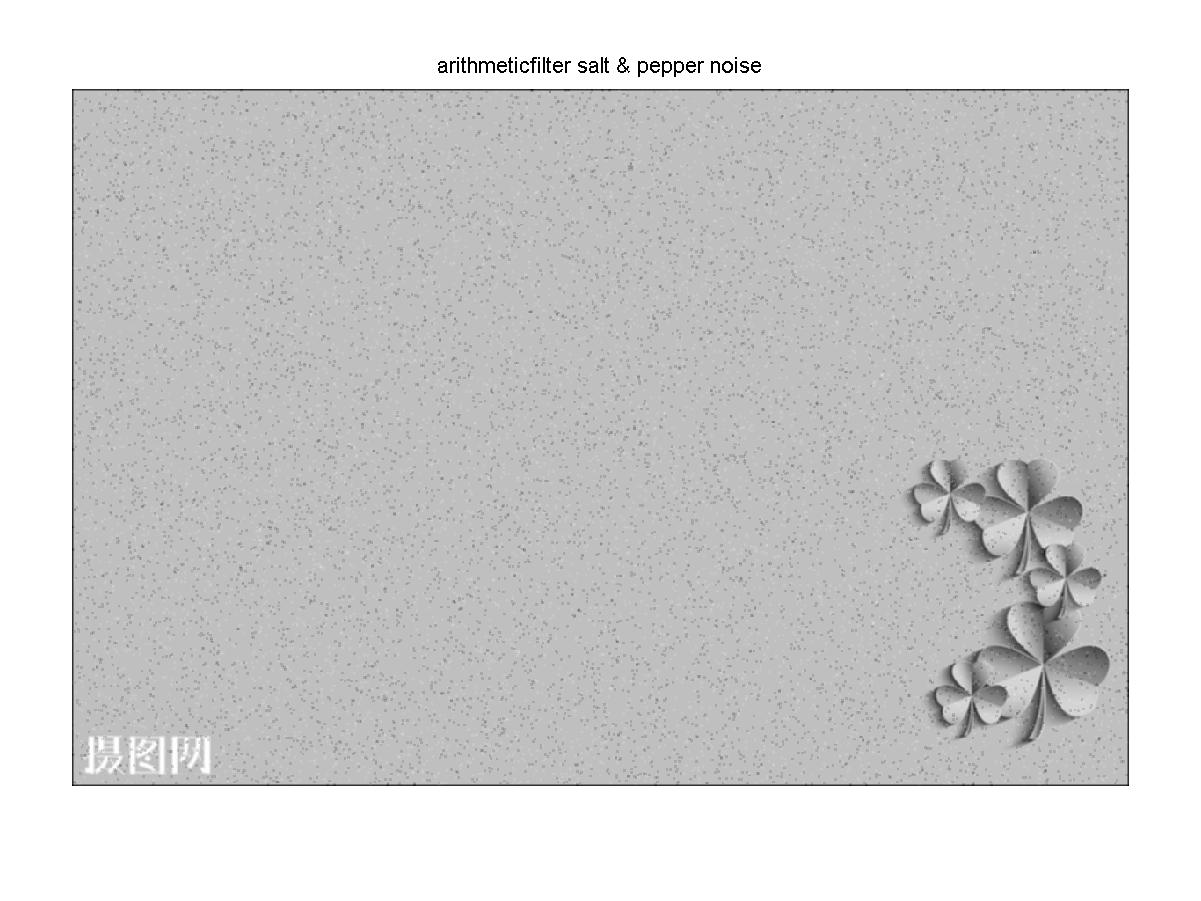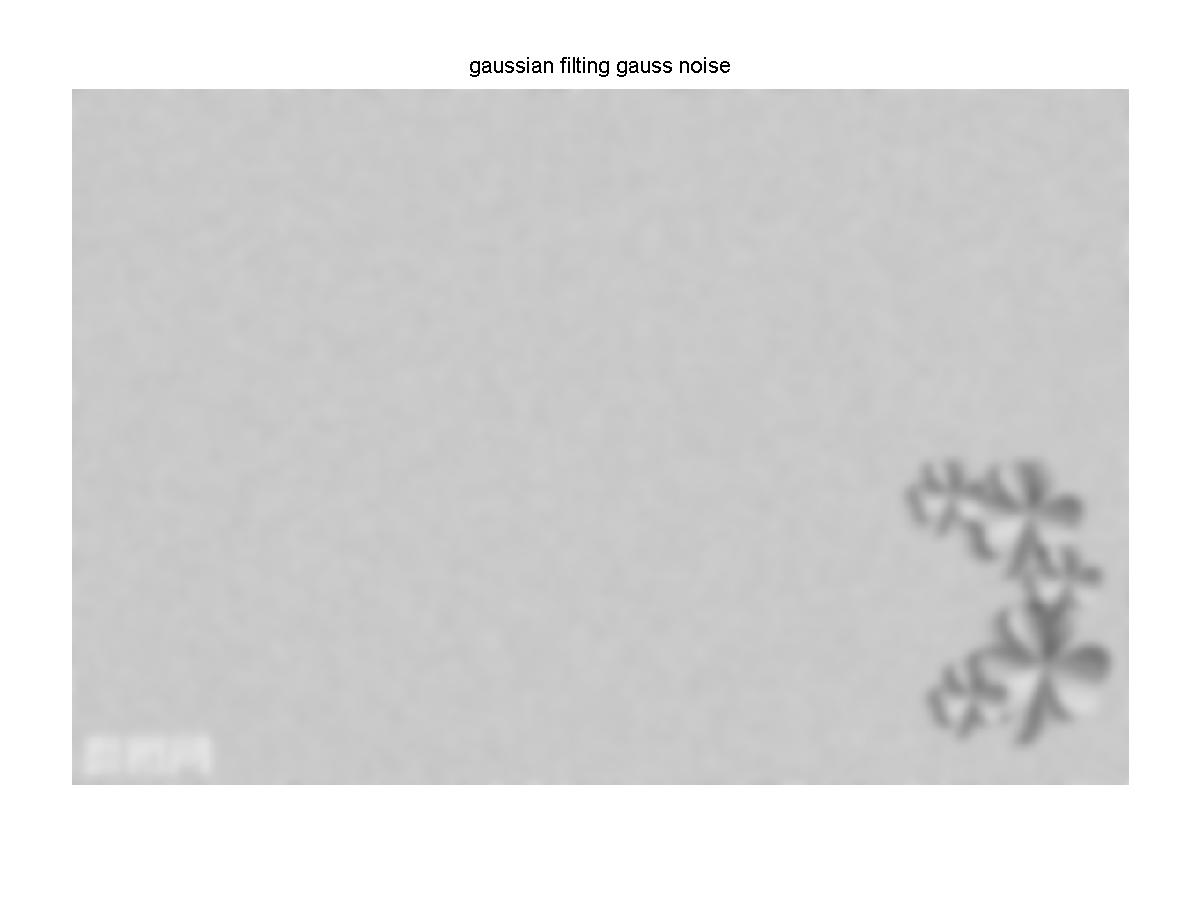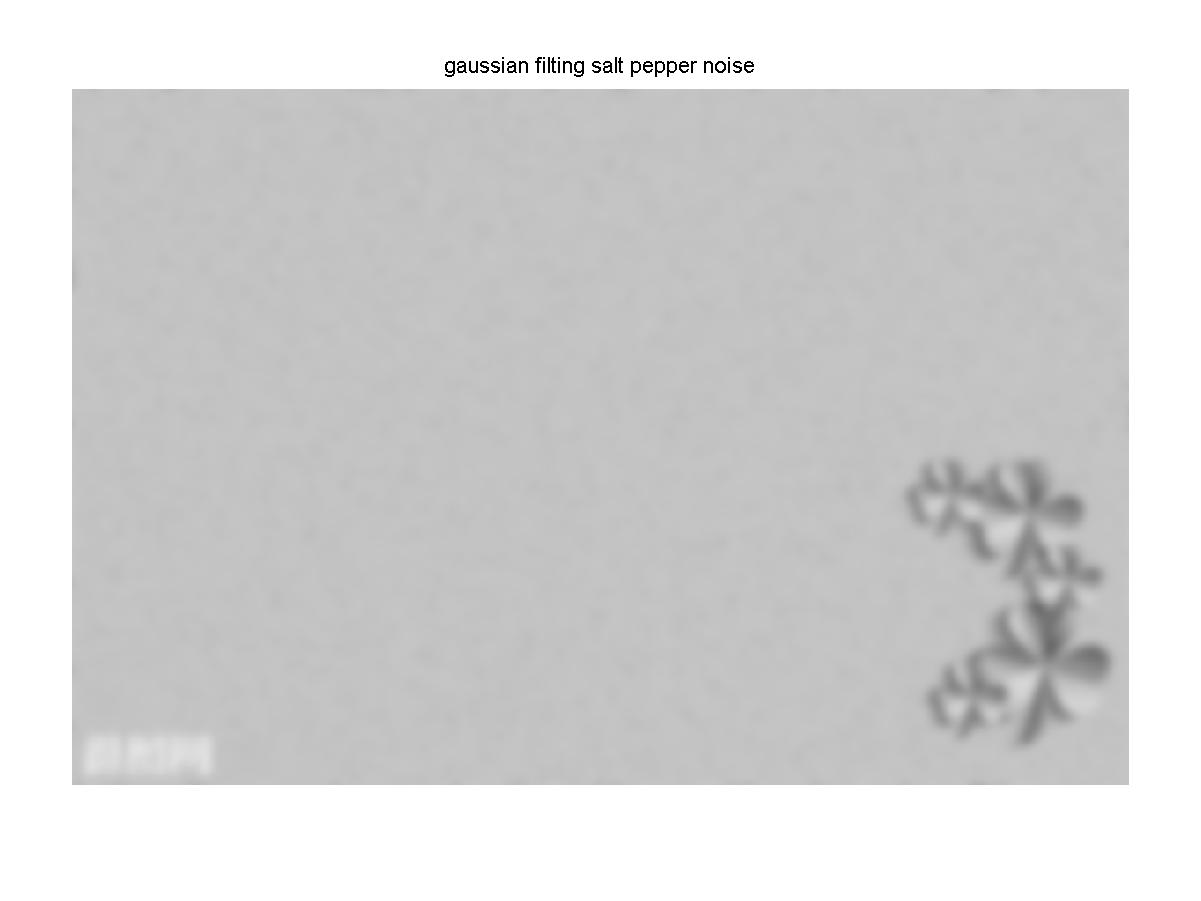FPGA自习学院将不断更新和总结FPGA相关学习资料，书籍，实例工程和视频。